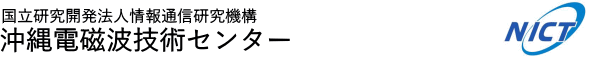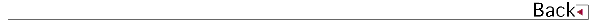\$B!!(B

# 2018\$BG/EY(B (2018.04\$B!A(B2019.03)

## \$B8&5fO@J8(B

• \$B?73@(B \$B5HLi(B, \$B1+C+(B \$B=c(B, Detection of GPS signal scattered from mountain. IEICE Communications Express,Vol.7 No.4 PP115-119

## \$B0lHL8}F,H/I=(B

• \$B;3K\(B \$B??G7(B, \$B@nB<(B \$B@?<#(B, \$B@>B<(B \$B9L;J(B, \$B?yC+(B \$BLPIW(B, \$B1+C+(B \$B=c(B, \$B;38}(B \$B90@?(B, \$BCfKL(B \$B1Q0l(B, \$B9bJ,2rG=(B1.3GHz\$BBS%&%#%s%I%W%m%U%!%\$%i\$N3+H/(B, \$BF|K\CO5eOG@12J3XO"9g(B2018\$BG/Bg2q(B
• \$B?73@(B \$B5HLi(B, \$B1+C+(B \$B=c(B, \$B?yC+(B \$BLPIW(B, \$B%G%8%?%k%F%l%S?.9f\$K\$h\$k3\$LL;6Mp\$N8!=P(B, \$BF|K\3\$MN3X2q(B
• \$B;T@n(B \$BN40l(B, \$B@.ED(B \$B=(\$B!!!!!!!!!!(B
• \$B;3K\(B \$B??G7(B, \$B@nB<(B \$B@?<#(B, \$B@>B<(B \$B9L;J(B, \$B?yC+(B \$BLPIW(B, \$B1+C+(B \$B=c(B, \$B;38}(B \$B90@?(B, \$BCfKL(B \$B1Q0l(B, \$B9bJ,2rG=(B1.3GHz\$BBS%&%#%s%I%W%m%U%!%\$%i\$N3+H/(B, Asia Oceania Geosciences Society (AOGS) 15th Annual Meeting
• \$B;T@n(B \$BN40l(B, \$B;a86(B \$B=(>Eg(B \$B7r(B, \$BBm8}(B \$BGn;N(B, \$B>>Eg(B \$B4nM:(B, \$BEmC+(B \$BC\$Li(B, \$B
• \$B;3K\(B \$B??G7(B, \$B@nB<(B \$B@?<#(B, \$B@>B<(B \$B9L;J(B, \$B?yC+(B \$BLPIW(B, \$B1+C+(B \$B=c(B, \$B;38}(B \$B90@?(B, \$BCfKL(B \$B1Q0l(B, \$B]3X2q(B2018\$BG/=)5(Bg2q(B\$B!!!!!!!!!!!!!!(B
• \$B?73@(B \$B5HLi(B, \$B1J9>(B \$BMW(B, \$BIMHf2E(B \$B@5=T(B, \$BF#0f(B \$BCR;K(B, \$B1+C+(B \$B=c(B, \$BCO%G%8%Q%C%7%V%l!<%@\$K\$h\$k1h4_N.B,Dj\$N2DG=@-(B, \$BBh#32s\$*\$-\$J\$o%^%j%s%5%\$%(%s%9%o!<%/%7%g%C%W(B
• \$B;T@n(B \$BN40l(B, \$B;a86(B \$B=( \$B!!!!!!!!!!!!!!(B
• \$B1J9>(B \$BMW(B, \$BIMHf2E(B \$B@5=T(B, \$B1+C+(B \$B=c(B, \$B?73@(B \$B5HLi(B, \$BF#0f(B \$BCR;K(B, \$BCO%G%8%Q%C%7%V%l!<%@\$K\$h\$k1h4_N.\$N8!=P(B, \$B3\$MN%l!<%@8&5f=82q!V3\$MN%l!<%@\$rMQ\$\$\$?3\$674F;k%7%9%F%`\$N3+H/\$H1~MQ!W(B

## 1990\$B!A(B1991\$B!d(B1992\$B!d(B1993\$B!d(B1994\$B!d(B1995\$B!d(B1996\$B!d(B1997\$B!d(B1998\$B!d(B1999\$B!d(B 2000\$B!d(B2001\$B!d(B2002\$B!d(B2003\$B!d(B2004\$B!d(B2005\$B!d(B2006\$B!d(B2007\$B!d(B2008\$B!d(B2009\$B!d(B 2010\$B!d(B2011\$B!d(B2012\$B!d(B2013\$B!d(B2014\$B!d(B2015\$B!d(B2016\$B!d(B2017> 2018